Скачать презентацию Loudness October 17 2007 What is

9cf96beed80c867e49acf1dd209432e6.ppt

• Количество слайдов: 34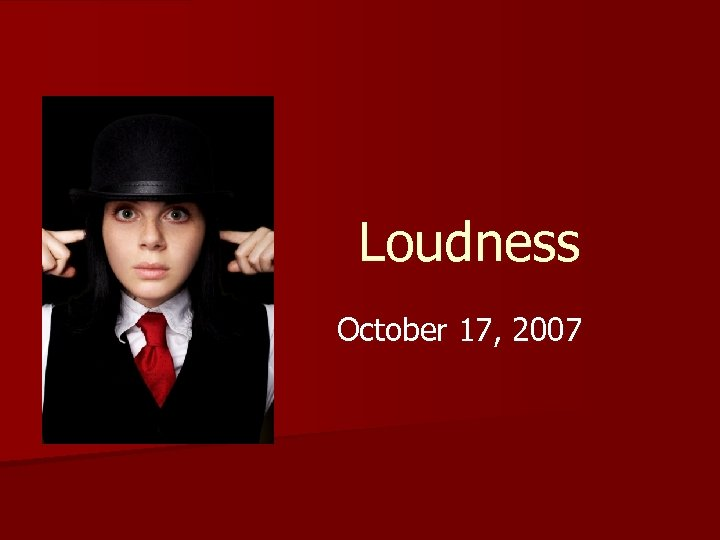Loudness October 17, 2007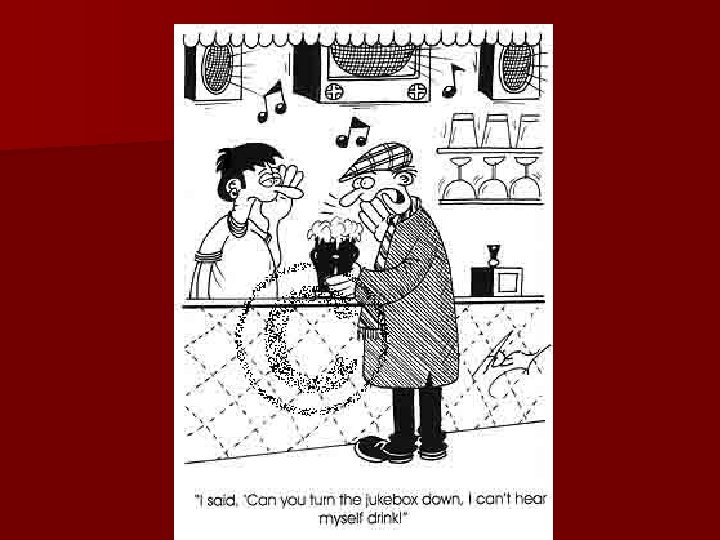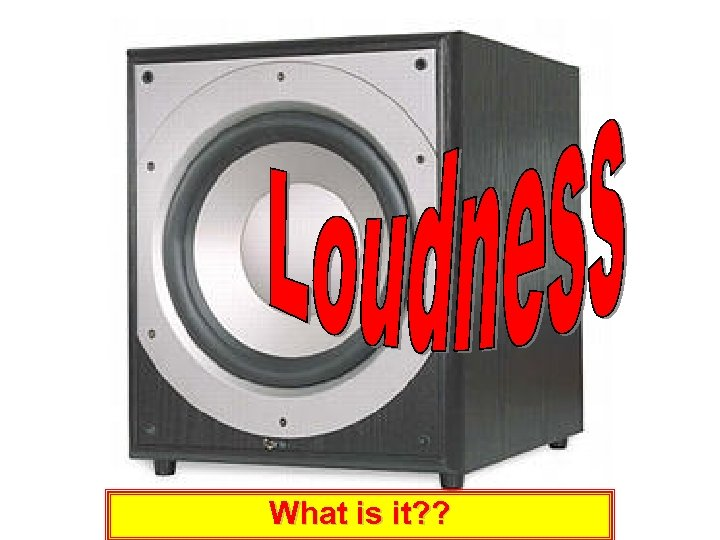What is it? ?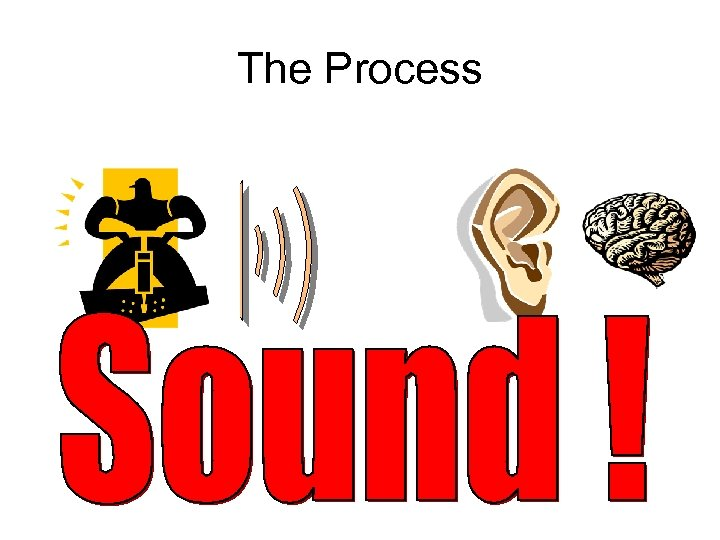The Process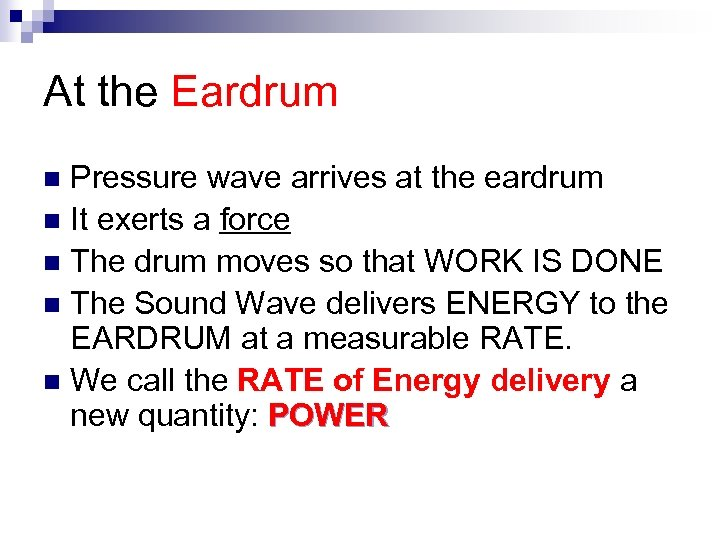At the Eardrum Pressure wave arrives at the eardrum n It exerts a force n The drum moves so that WORK IS DONE n The Sound Wave delivers ENERGY to the EARDRUM at a measurable RATE. n We call the RATE of Energy delivery a new quantity: POWER n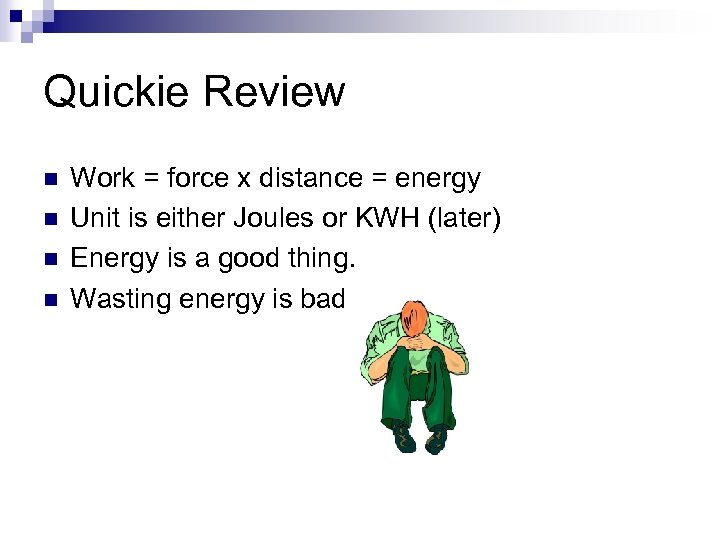Quickie Review n n Work = force x distance = energy Unit is either Joules or KWH (later) Energy is a good thing. Wasting energy is bad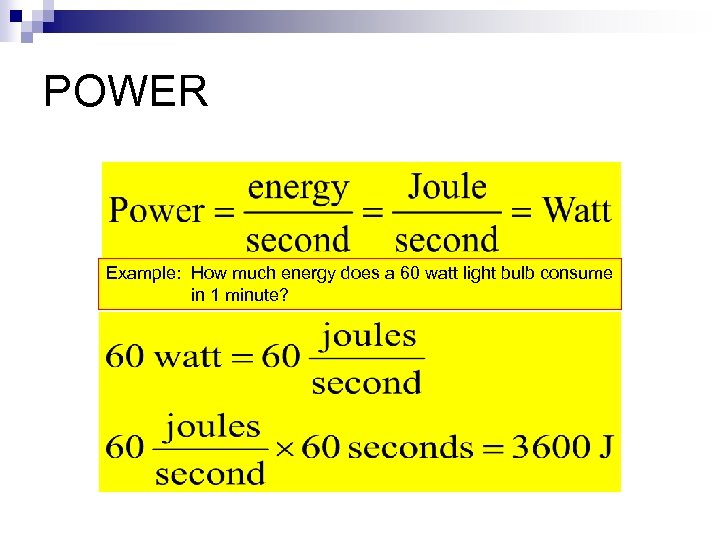POWER Example: How much energy does a 60 watt light bulb consume in 1 minute?By the way …. . You BUY KWH from the power company.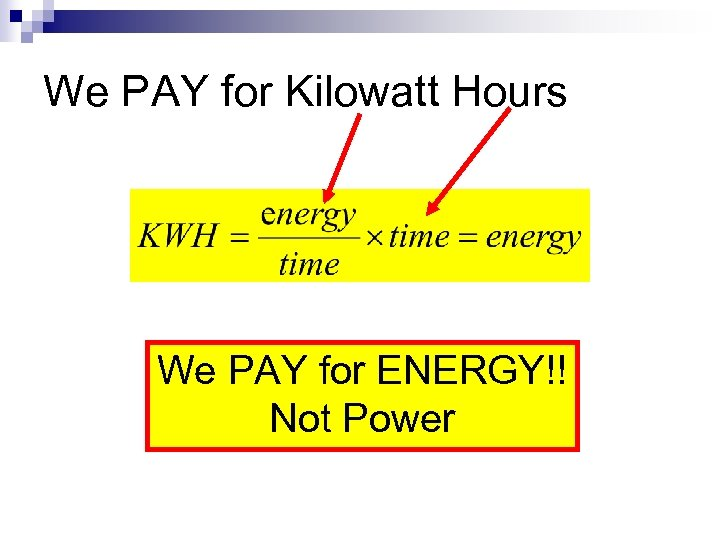We PAY for Kilowatt Hours We PAY for ENERGY!! Not Power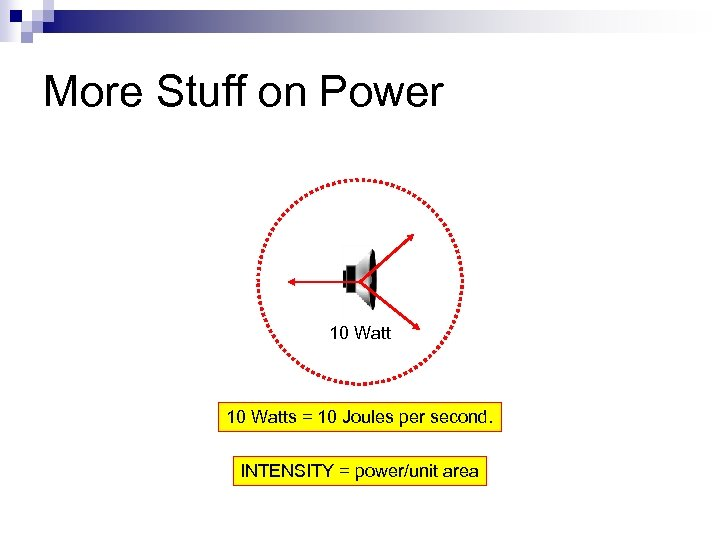More Stuff on Power 10 Watts = 10 Joules per second. INTENSITY = power/unit area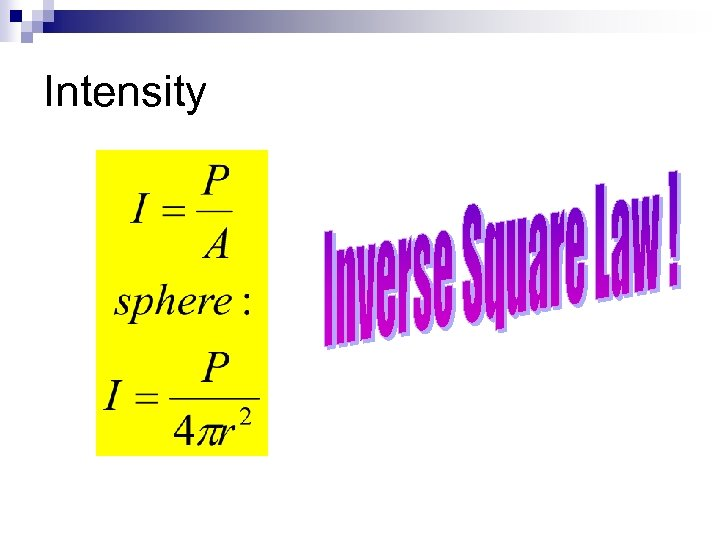Intensity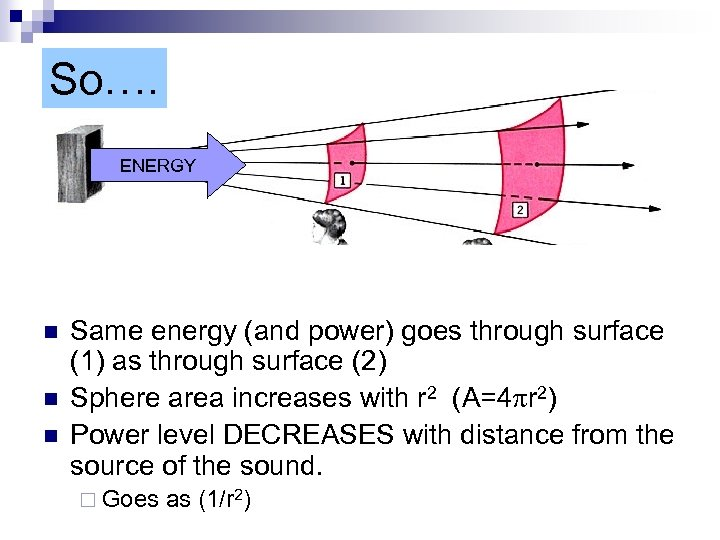So…. ENERGY n n n Same energy (and power) goes through surface (1) as through surface (2) Sphere area increases with r 2 (A=4 pr 2) Power level DECREASES with distance from the source of the sound. ¨ Goes as (1/r 2)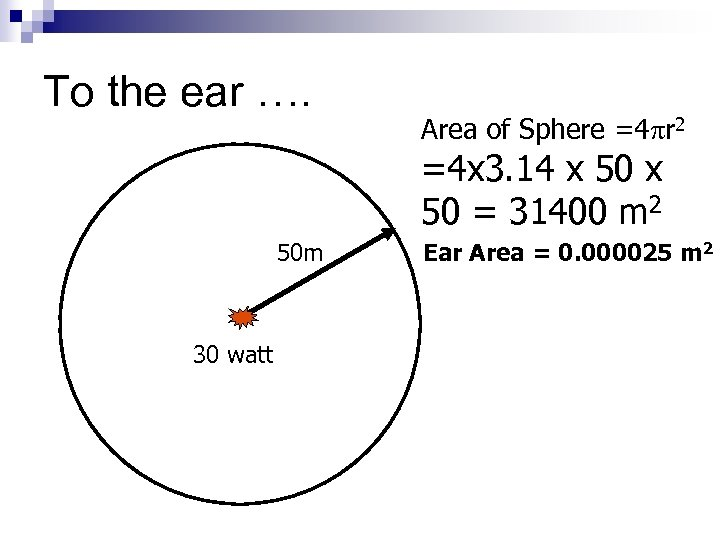To the ear …. Area of Sphere =4 pr 2 =4 x 3. 14 x 50 = 31400 m 2 50 m 30 watt Ear Area = 0. 000025 m 2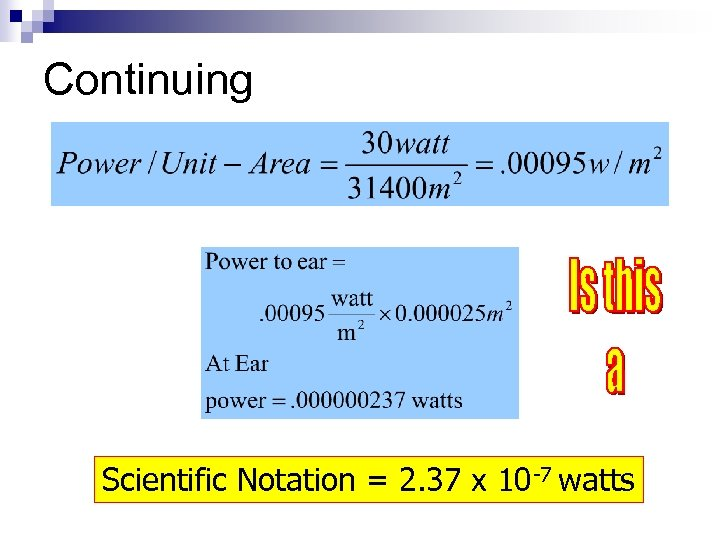Continuing Scientific Notation = 2. 37 x 10 -7 watts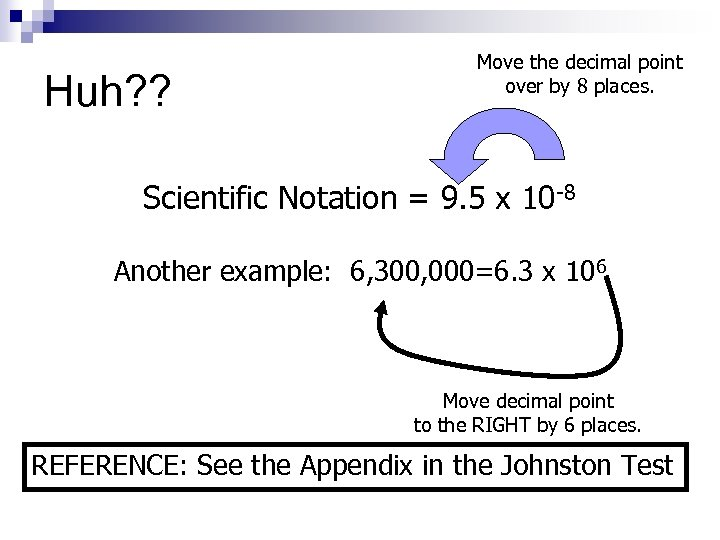Huh? ? Move the decimal point over by 8 places. Scientific Notation = 9. 5 x 10 -8 Another example: 6, 300, 000=6. 3 x 106 Move decimal point to the RIGHT by 6 places. REFERENCE: See the Appendix in the Johnston Test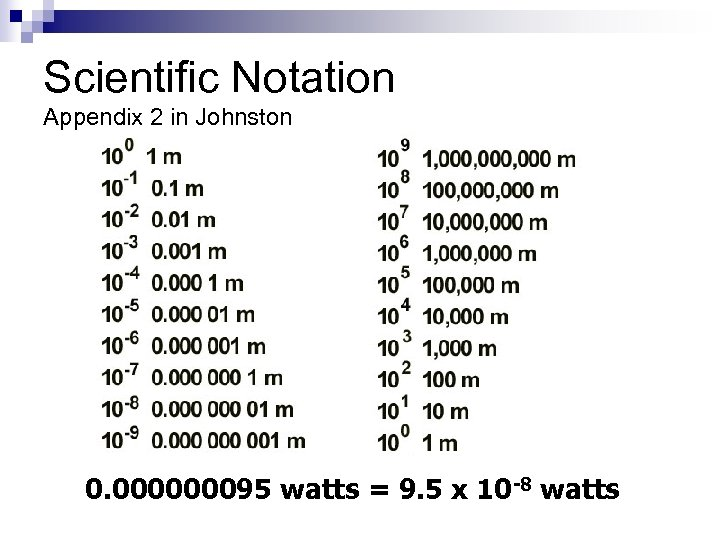Scientific Notation Appendix 2 in Johnston 0. 000000095 watts = 9. 5 x 10 -8 watts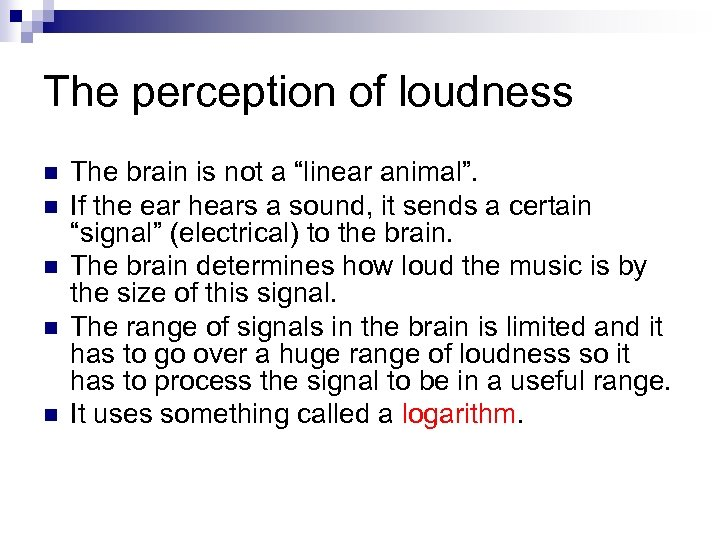The perception of loudness n n n The brain is not a “linear animal”. If the ear hears a sound, it sends a certain “signal” (electrical) to the brain. The brain determines how loud the music is by the size of this signal. The range of signals in the brain is limited and it has to go over a huge range of loudness so it has to process the signal to be in a useful range. It uses something called a logarithm.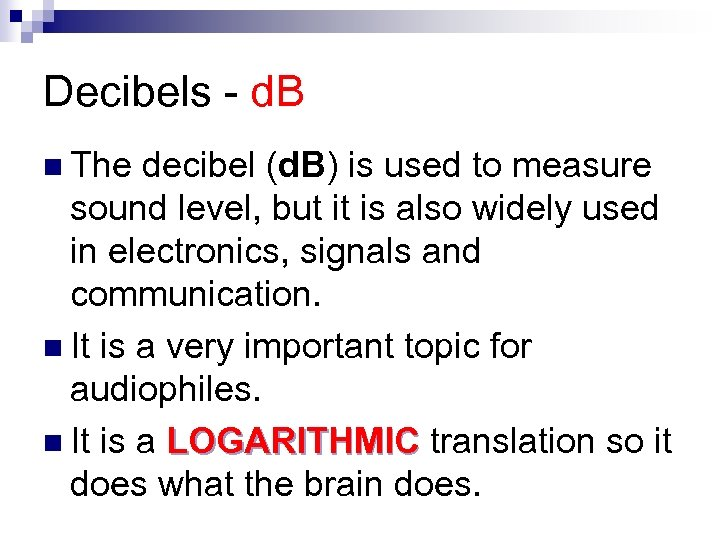Decibels - d. B n The decibel (d. B) is used to measure sound level, but it is also widely used in electronics, signals and communication. n It is a very important topic for audiophiles. n It is a LOGARITHMIC translation so it does what the brain does.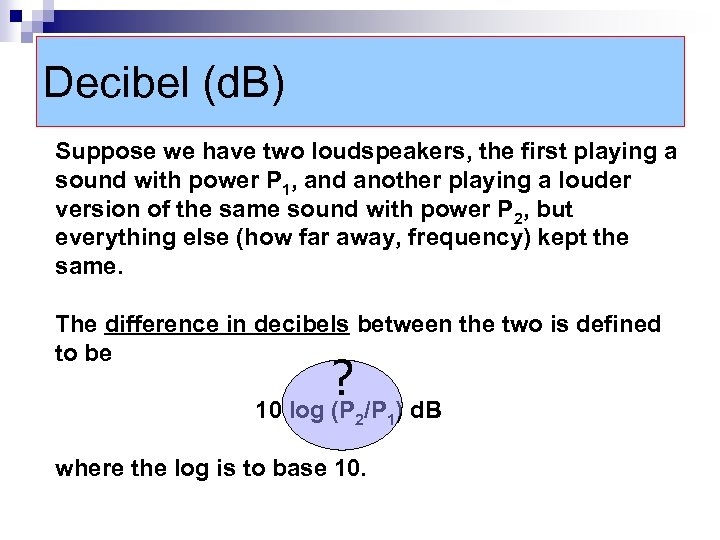Decibel (d. B) Suppose we have two loudspeakers, the first playing a sound with power P 1, and another playing a louder version of the same sound with power P 2, but everything else (how far away, frequency) kept the same. The difference in decibels between the two is defined to be ? /P ) d. B 10 log (P 2 where the log is to base 10. 1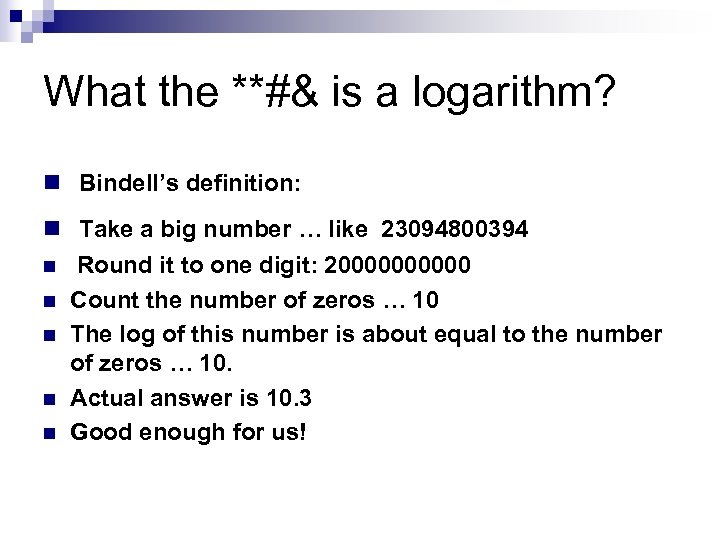What the **#& is a logarithm? n Bindell’s definition: n Take a big number … like 23094800394 n Round it to one digit: 200000 n Count the number of zeros … 10 n The log of this number is about equal to the number of zeros … 10. n Actual answer is 10. 3 n Good enough for us!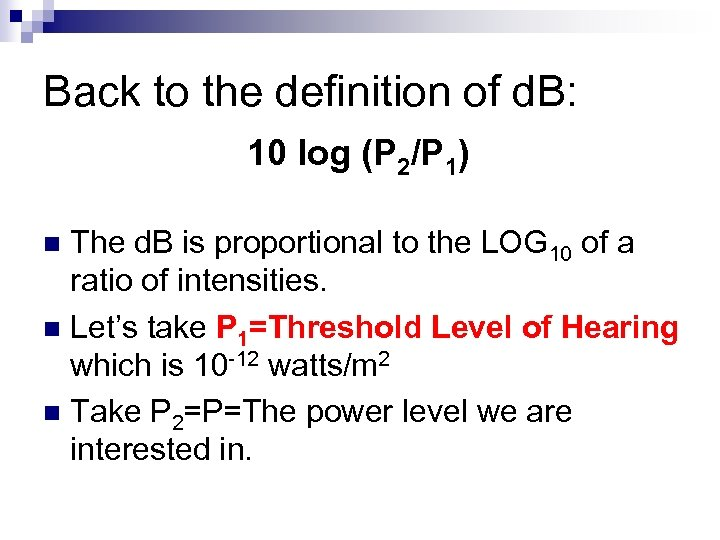Back to the definition of d. B: 10 log (P 2/P 1) The d. B is proportional to the LOG 10 of a ratio of intensities. n Let’s take P 1=Threshold Level of Hearing which is 10 -12 watts/m 2 n Take P 2=P=The power level we are interested in. n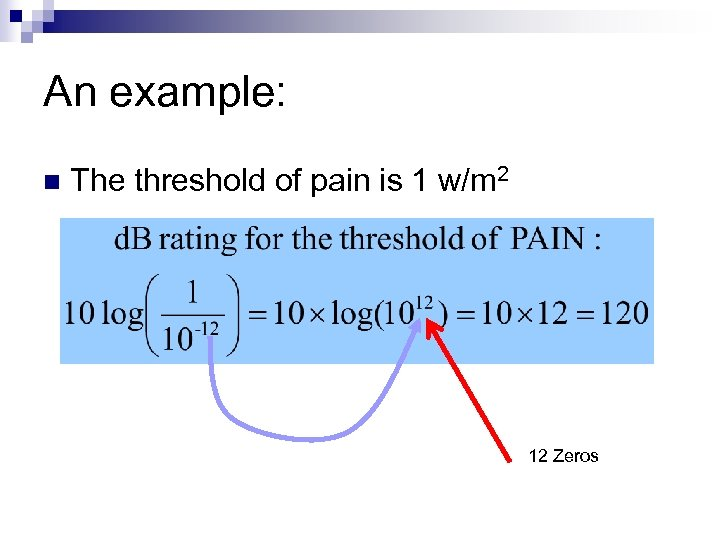An example: n The threshold of pain is 1 w/m 2 12 Zeros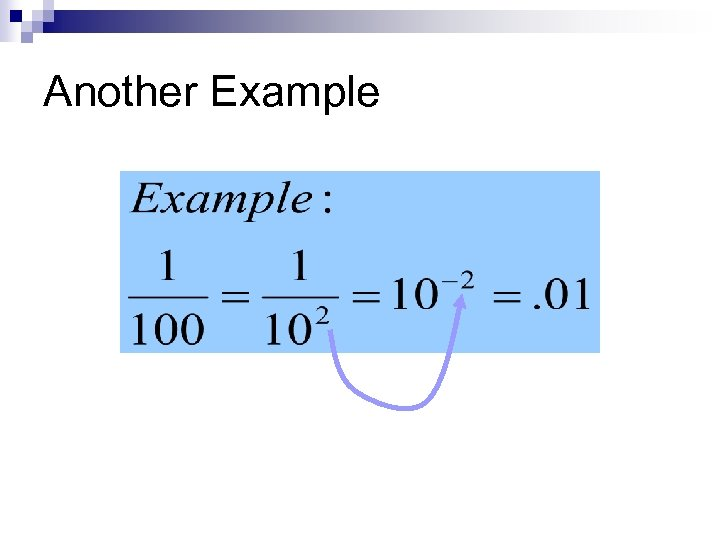Another Example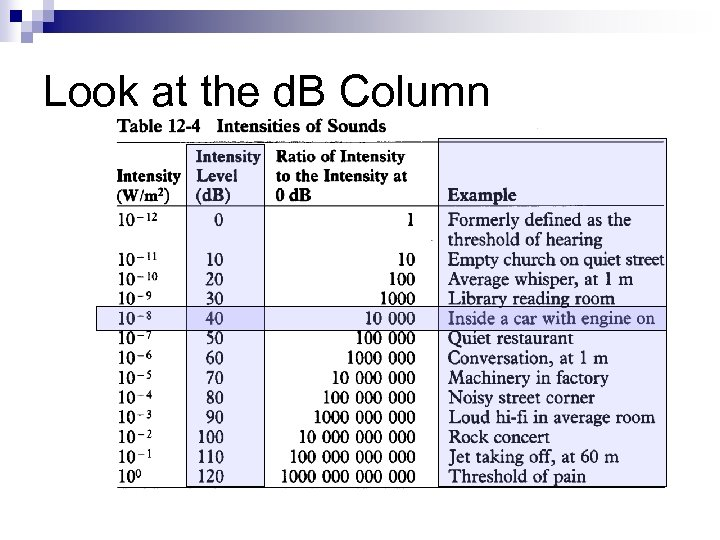Look at the d. B Column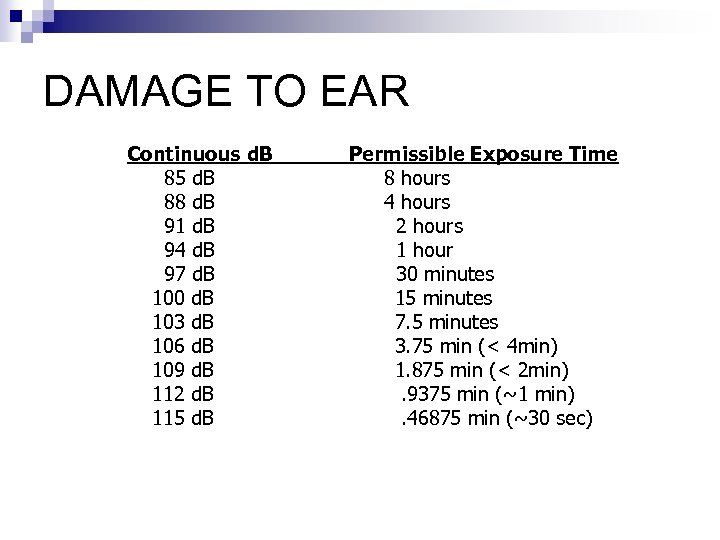DAMAGE TO EAR Continuous d. B Permissible Exposure Time 85 d. B 8 hours 88 d. B 4 hours 91 d. B 2 hours 94 d. B 1 hour 97 d. B 30 minutes 100 d. B 15 minutes 103 d. B 7. 5 minutes 106 d. B 3. 75 min (< 4 min) 109 d. B 1. 875 min (< 2 min) 112 d. B . 9375 min (~1 min) 115 d. B . 46875 min (~30 sec)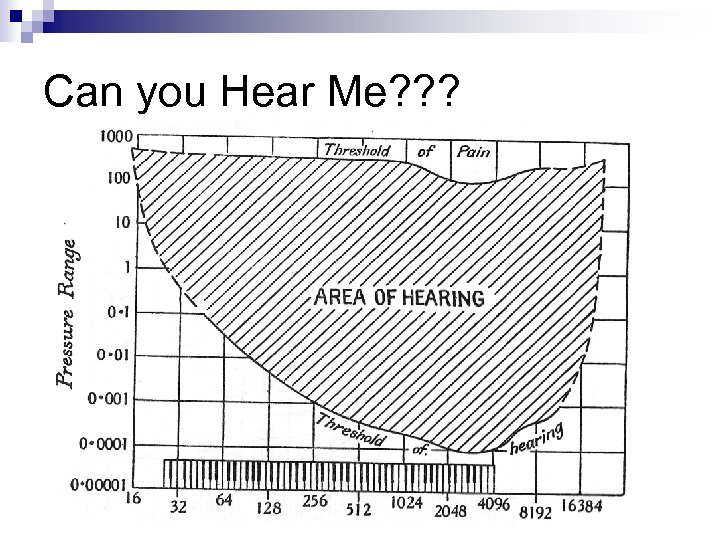Can you Hear Me? ? ?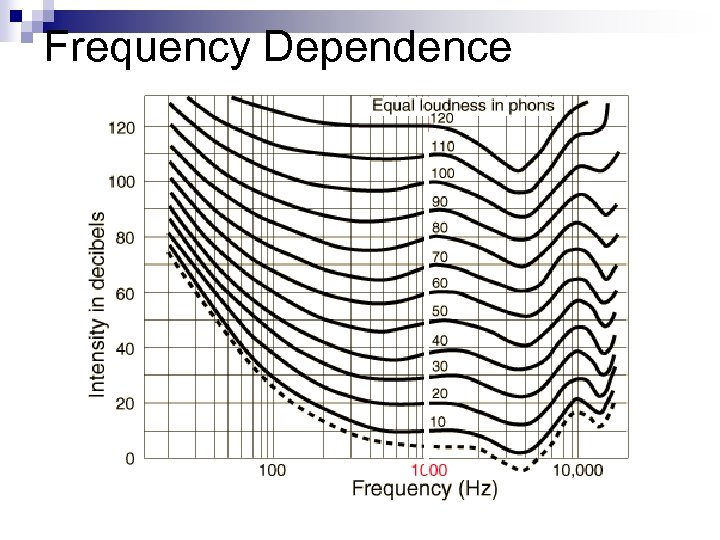Frequency Dependence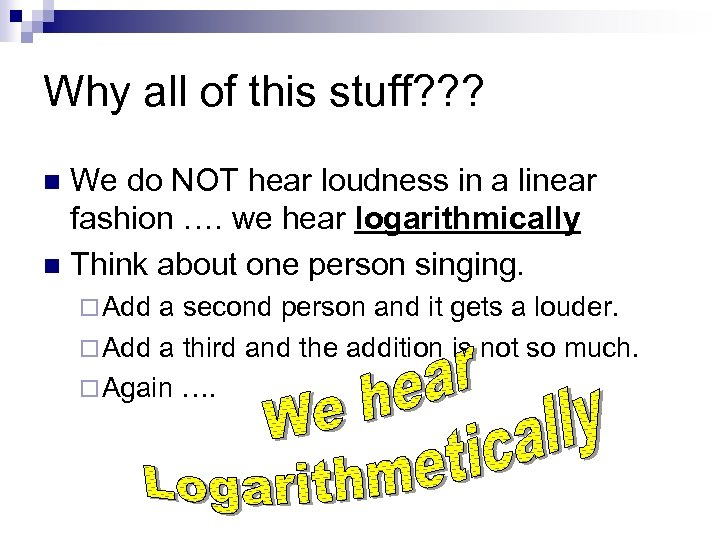Why all of this stuff? ? ? We do NOT hear loudness in a linear fashion …. we hear logarithmically n Think about one person singing. n ¨ Add a second person and it gets a louder. ¨ Add a third and the addition is not so much. ¨ Again ….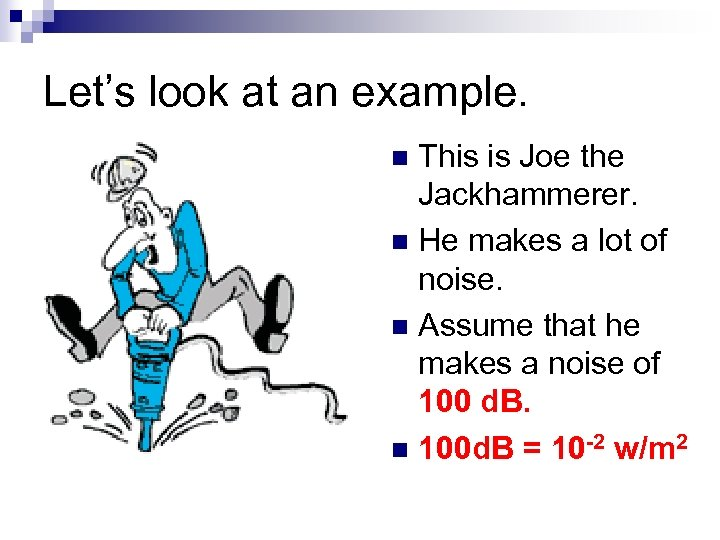Let’s look at an example. This is Joe the Jackhammerer. n He makes a lot of noise. n Assume that he makes a noise of 100 d. B. n 100 d. B = 10 -2 w/m 2 n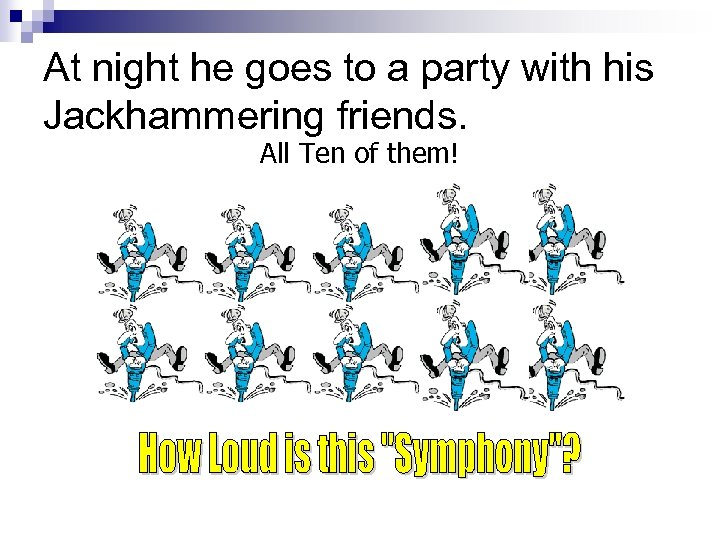At night he goes to a party with his Jackhammering friends. All Ten of them!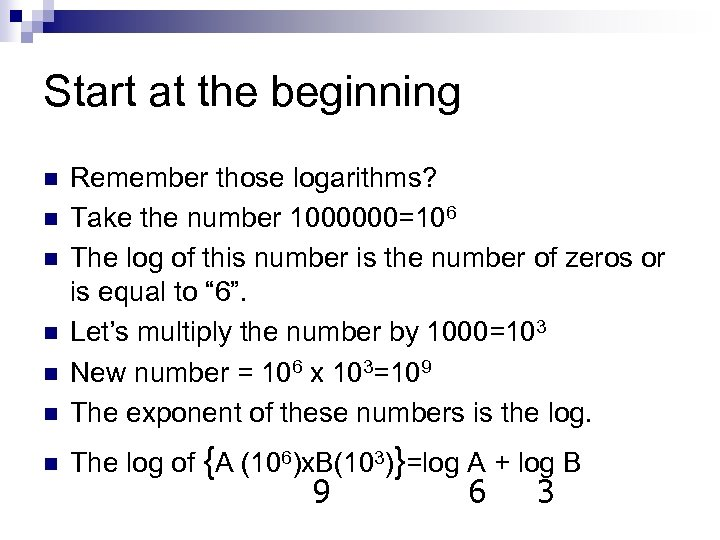Start at the beginning n Remember those logarithms? Take the number 1000000=106 The log of this number is the number of zeros or is equal to “ 6”. Let’s multiply the number by 1000=103 New number = 106 x 103=109 The exponent of these numbers is the log. n The log of {A (106)x. B(103)}=log A + log B n n n 9 6 3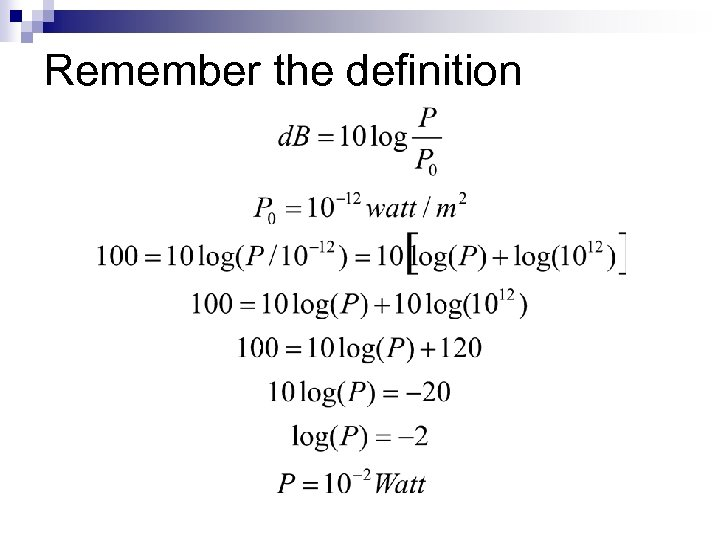Remember the definition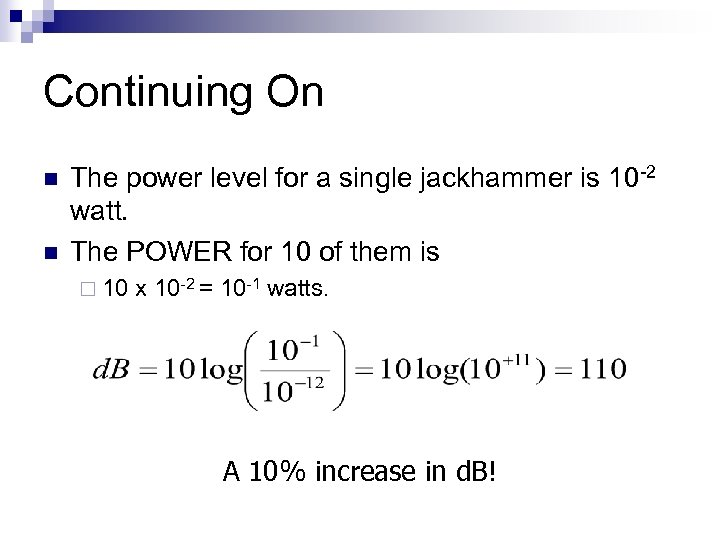Continuing On n n The power level for a single jackhammer is 10 -2 watt. The POWER for 10 of them is ¨ 10 x 10 -2 = 10 -1 watts. A 10% increase in d. B!Let’s YELL!!!!